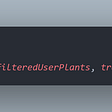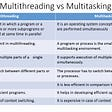# 12/20/2020

Note:

2. The solutions are coded by myself, and open source just for study.

Refer to the Oracle Help Cente, the comparator is a functional interface that can be used as an assignment target for a lambda expression or method interface. That means, we need to implement it in our script.

We’ve already had the basic structure of this problem. Looking at the “Player”, we have a name and score, which can be compared in the “Checker” method. Let’s figure it out now.

`import java.util.*;// Write your Checker class hereclass Checker implements Comparator<Player>{    @Override    public int compare(Player p1, Player p2){        if (p1.score == p2.score){            return p1.name.compareTo(p2.name);        }        else {            return p2.score - p1.score;        }    }}class Player{    String name;    int score;    Player(String name, int score){        this.name = name;        this.score = score;    }}class Solution {    public static void main(String[] args) {        Scanner scan = new Scanner(System.in);        int n = scan.nextInt();        Player[] player = new Player[n];        Checker checker = new Checker();        for(int i = 0; i < n; i++){            player[i] = new Player(scan.next(), scan.nextInt());        }        scan.close();        Arrays.sort(player, checker);        for(int i = 0; i < player.length; i++){            System.out.printf("%s %s\n", player[i].name, player[i].score);        }    }}`

It worked and it should not be difficult to understand. Let’s move on!

(Actually, I should work on the Java Deque problem first, but I did not figure out how to solve it yet. So, let’s pick an easier one)

Well, before that, let’s figure out: what is Vararg (variable arguments) in Java?

Refer to this doc, Vararg can be called with zero or more arguments. Is implicitly declared as an array of type int . Sounds good in this question, right?

The initial try is as below:

`import java.io.*;import java.lang.reflect.*;import java.util.*;import java.text.*;import java.math.*;import java.util.regex.*;//Write your code hereclass Add {    public void add(int... Args) {        int sum = 0;        for (int i : Args) {            sum += i;            System.out.print(i + "+");        }        System.out.println("=" + sum);    }}public class Solution {    public static void main(String[] args) {        try{            BufferedReader br=new BufferedReader(new InputStreamReader(System.in));            int n1=Integer.parseInt(br.readLine());            int n2=Integer.parseInt(br.readLine());            int n3=Integer.parseInt(br.readLine());            int n4=Integer.parseInt(br.readLine());            int n5=Integer.parseInt(br.readLine());            int n6=Integer.parseInt(br.readLine());            Add ob=new Add();            ob.add(n1,n2);            ob.add(n1,n2,n3);            ob.add(n1,n2,n3,n4,n5);            ob.add(n1,n2,n3,n4,n5,n6);            Method[] methods=Add.class.getDeclaredMethods();            Set<String> set=new HashSet<>();            boolean overload=false;            for(int i=0;i<methods.length;i++)            {                if(set.contains(methods[i].getName()))                {                    overload=true;                    break;                }                set.add(methods[i].getName());            }            if(overload)            {                throw new Exception("Overloading not allowed");            }        }        catch(Exception e)        {            e.printStackTrace();        }    }}`

Unfortunately, it failed all tests. What’s wrong with it?

I referred to the discussion board and found we may need a string to separate them. Here is the edited version:

`import java.io.*;import java.lang.reflect.*;import java.util.*;import java.text.*;import java.math.*;import java.util.regex.*;//Write your code hereclass Add {    public void add(int... Args) {        int sum = 0;        String s = "";        for (int i : Args) {            sum += i;            System.out.print(s + i);            s = "+";        }        System.out.println("=" + sum);    }}public class Solution {    public static void main(String[] args) {        try{            BufferedReader br=new BufferedReader(new InputStreamReader(System.in));            int n1=Integer.parseInt(br.readLine());            int n2=Integer.parseInt(br.readLine());            int n3=Integer.parseInt(br.readLine());            int n4=Integer.parseInt(br.readLine());            int n5=Integer.parseInt(br.readLine());            int n6=Integer.parseInt(br.readLine());            Add ob=new Add();            ob.add(n1,n2);            ob.add(n1,n2,n3);            ob.add(n1,n2,n3,n4,n5);            ob.add(n1,n2,n3,n4,n5,n6);            Method[] methods=Add.class.getDeclaredMethods();            Set<String> set=new HashSet<>();            boolean overload=false;            for(int i=0;i<methods.length;i++)            {                if(set.contains(methods[i].getName()))                {                    overload=true;                    break;                }                set.add(methods[i].getName());            }            if(overload)            {                throw new Exception("Overloading not allowed");            }        }        catch(Exception e)        {            e.printStackTrace();        }    }}`

It passed! Cheers!

(To be honest, I think the body of this problem can be more concise and decent, but I did not try to upgrade it. Hope we may have a chance in the future!)

I don’t know how to describe this problem, but the idea is explicit. We should use some method to retrieve public class, and it is “.getDeclaredMethod()” in the statement. The code is as below:

`public class Solution {    public static void main(String[] args){        Class student = Student.class;        Method[] methods = student.getDeclaredMethods();        ArrayList<String> methodList = new ArrayList<>();        for(Method name: methods){            methodList.add(name.getName());        }        Collections.sort(methodList);        for(String name: methodList){            System.out.println(name);        }    }}`

Noteworthy, the IDE of Hacker Rank is a little bit different this time. I assume that’s because we need embedded classes this time (the “Student” class). And the code worked. Woo!

This is not difficult! We just need to check the classes and implement them in this interface. The basic structure is already provided. Let’s have a try:

`import java.util.*;import java.security.*;interface Food {    public String getType();}class Pizza implements Food {    public String getType() {        return "Someone ordered a Fast Food!";    }}class Cake implements Food {    public String getType() {        return "Someone ordered a Dessert!";    }}class FoodFactory {    public Food getFood(String order) {        //Write your code here        if (order.equals("pizza")){            return new Pizza();        }        else if (order.equals("cake")){            return new Cake();        }    }//End of getFood method}//End of factory classpublic class Solution {    public static void main(String args[]){        Do_Not_Terminate.forbidExit();        try{            Scanner sc=new Scanner(System.in);            //creating the factory            FoodFactory foodFactory = new FoodFactory();            //factory instantiates an object            Food food = foodFactory.getFood(sc.nextLine());            System.out.println("The factory returned "+food.getClass());            System.out.println(food.getType());        }        catch (Do_Not_Terminate.ExitTrappedException e) {            System.out.println("Unsuccessful Termination!!");        }    }}class Do_Not_Terminate {    public static class ExitTrappedException extends SecurityException {        private static final long serialVersionUID = 1L;    }    public static void forbidExit() {        final SecurityManager securityManager = new SecurityManager() {            @Override            public void checkPermission(Permission permission) {                if (permission.getName().contains("exitVM")) {                    throw new ExitTrappedException();                }            }        };        System.setSecurityManager(securityManager);    }}`

Unfortunately, this failed. It says we have no “return” in this method, what should we do?

After adding “return null,” the code is like this:

`import java.util.*;import java.security.*;interface Food {    public String getType();}class Pizza implements Food {    public String getType() {        return "Someone ordered a Fast Food!";    }}class Cake implements Food {    public String getType() {        return "Someone ordered a Dessert!";    }}class FoodFactory {    public Food getFood(String order) {        //Write your code here        if (order.equals("pizza")){            return new Pizza();        }        else if (order.equals("cake")){            return new Cake();        }        return null;    }//End of getFood method}//End of factory classpublic class Solution {    public static void main(String args[]){        Do_Not_Terminate.forbidExit();        try{            Scanner sc=new Scanner(System.in);            //creating the factory            FoodFactory foodFactory = new FoodFactory();            //factory instantiates an object            Food food = foodFactory.getFood(sc.nextLine());            System.out.println("The factory returned "+food.getClass());            System.out.println(food.getType());        }        catch (Do_Not_Terminate.ExitTrappedException e) {            System.out.println("Unsuccessful Termination!!");        }    }}class Do_Not_Terminate {    public static class ExitTrappedException extends SecurityException {        private static final long serialVersionUID = 1L;    }    public static void forbidExit() {        final SecurityManager securityManager = new SecurityManager() {            @Override            public void checkPermission(Permission permission) {                if (permission.getName().contains("exitVM")) {                    throw new ExitTrappedException();                }            }        };        System.setSecurityManager(securityManager);    }}`

It worked! Cheers!

I wanted to finish this section (Java certification on Hacker Rank) before 2021, but it seems not so easy to accomplish. Anyway, let’s keep moving!

References:

--

--

--

Undergraduate student / Research assistant/ Always curious / Opinions are mine

Love podcasts or audiobooks? Learn on the go with our new app.

## Making Sure Things Work (or PlantSwap Project Part 4)## Successful Documentation Strategy## Using Custom Font and Hindi Font in iText PDF Library.## Infracost : Cost estimates for the IaC Resources in Azure## How to get stars and be GitHub #1## Automation using Ansible, Configuring Docker## Your First Hybrid Ionic 5 App from Scratch.## Andrew Chen

Undergraduate student / Research assistant/ Always curious / Opinions are mine

## Add Superscript and Subscript to PowerPoint Using Java## Why public static void main(String args[]) | Java | Explained